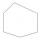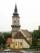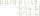# In a 2

In a thirteen sided polygon, the sum of five angles is 1274°, four of the eight angles remaining are equal and the other four are 18° less than each of the equal angles. Find the angles. .

Result

A =  97.25
B =  79.25

#### Solution:

1274 + 4A + 4B = 180*(13-2)
B = A - 18

4A+4B = 706
A-B = 18

A = 3894 = 97.25
B = 3174 = 79.25

Calculated by our linear equations calculator.

Leave us a comment of example and its solution (i.e. if it is still somewhat unclear...):

Showing 1 comment:Dr Math
A = 97.25 (4 angles)
B = 79.25  (last 4 angles)
first 5 angles cannot be determined#### To solve this example are needed these knowledge from mathematics:

Do you have a system of equations and looking for calculator system of linear equations? See also our trigonometric triangle calculator.

## Next similar examples:

1. Six-sided polygonIn a six-sided polygon. The first two angles are equal, the third angle is twice (the equal angles), two other angles are trice the equal angle, while the last angle is a right angle. Find the value of each angle.
2. Rotatable towerRotatable tower situated in the city center has ground shape of a regular polygon. If the tower is rotated by 14.4° around its centerpiece it looks from the side same. Your task is to calculate at least how many vertices can have a ground plan view of the.
3. Trapezium internal anglesA trapezium where AB is parallel to CD, has angle A : angle D = 4 :5, angle B = 3x-15 and angle C = 4x+20. Find angle A, B, C and D.
4. Triangle 42Triangle BCA. Angles A=119° B=(3y+14) C=4y. What is measure of triangle BCA=?
5. Angles in triangleThe triangle is ratio of the angles β:γ = 6:8. Angle α is 40° greater than β. What are the size of angles of the triangle?
6. A triangleA triangle has an angle that is 63.1 other 2 are in ratio of 2:5 What are the measurements of the two angles?
7. AnglesIn the triangle ABC, the ratio of angles is: a:b = 4: 5. The angle c is 36°. How big are the angles a, b?
8. Internal anglesFind the internal angles of the triangle ABC if the angle at the vertex C is twice the angle at the B and the angle at the vertex B is 4 degrees smaller than the angle at the vertex A.
9. Reference angleFind the reference angle of each angle:
10. Theorem proveWe want to prove the sentense: If the natural number n is divisible by six, then n is divisible by three. From what assumption we started?
11. Linsys2Solve two equations with two unknowns: 400x+120y=147.2 350x+200y=144
12. Three unknownsSolve the system of linear equations with three unknowns: A + B + C = 14 B - A - C = 4 2A - B + C = 0
13. Three workshopsThere are 2743 people working in three workshops. In the second workshop works 140 people more than in the first and in third works 4.2 times more than the second one. How many people work in each workshop?
14. Inverse matrixFind out inverse by Gauss elimination or by reduction method. A=[2/3. 1 -3. 1/3]
15. Elimination methodSolve system of linear equations by elimination method: 5/2x + 3/5y= 4/15 1/2x + 2/5y= 2/15
16. AP - basicsDetermine first member and differentiate of the the following sequence: a3-a5=24 a4-2a5=61
17. Ball gameRichard, Denis and Denise together scored 932 goals. Denis scored 4 goals over Denise but Denis scored 24 goals less than Richard. Determine the number of goals for each player.